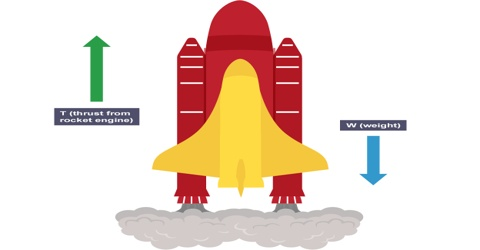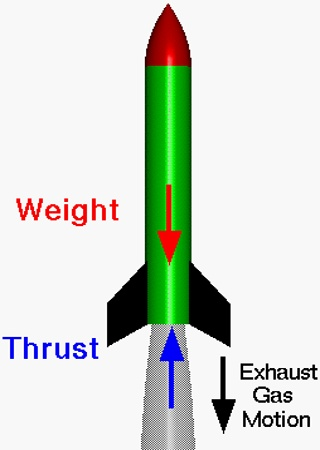Physics

# Applications of Newton’s Laws of Motion: Exploration in the SpaceApplications of Newton’s Laws of Motion

When a body applies force on another body then the second body also applies equal and opposite force on the first body. We have learnt about this action and reaction forces from Newton’s third law of motion. In nature forces act in couples. In nature there is not individual separate force. Two forces are complementary to each other. One of these forces is called action force and the other one is reaction force. As long as action force is there, there exists reaction force also. Practical application of Newton’s laws of motion is described below with example.

Exploration in the space:

When a rocket, during exploration in the sky, moves upward, then if you look at the rocket you will see that smoke like white cloud comes out through the backward nozzles. Can you say why such smoke is seen? Due to burning of fuel gas is formed at very high pressure. We often see this coil of gas from the earth. This gas comes out through the small opening at the rear side of the rocket with tremendous velocity. Due to this, a tremendous reaction force is produced which pushes the rocket forward with a very strong velocity [Figure].Extensive uses of artificial satellites are found in modern telecommunication system and artificial satellites have also great contribution to space research. Behind this successive development of rocket technology is playing a vital role.

Although gas is lighter but due to the very high-velocity momentum of the emitted gas is very high. According to conservation principle of momentum the rocket also acquires equal but oppositely directed momentum and hence rises upward with high velocity. Normally, as fuel rocket uses liquid hydrogen and for ignition liquid oxygen is used. By a special process and controlled rate liquid hydrogen and oxygen are allowed to enter into the combustion chamber. Due to the burning of the fuel high pressure gas is produced that comes out at a very high speed through the opening at the bottom at the rocket.

Let consider that a rocket is in motion in the space. So we can ignore air resistance and influence of gravity. Since due to the emission of gas from the rocket a force or a thrust against the motion of the gas is generated this pushes the rocket ahead with a very high speed. By the help of rocket, it has been possible to acquire the escape velocity (11.2 kms-1) and hence by overcoming the influence of acceleration due to gravity the satellites can be stationed in space and various explorations have been successful.

Let the applied thrust = F

Mass of the rocket = M

Mass of the ejected gas at time ∆t =  ∆m

Escape velocity of gas = v,

Change of momentum of the gas in time interval, ∆t = (∆m)v

According to conservation principle of momentum;

Change of momentum in time ∆t = applied impulse force on the rocket

so, (∆m)v = F x ∆t

F = (∆m/∆t) v; here, (∆m/∆t) = rate of using of fuel.

If the instantaneous acceleration of the rocket is a, then F = Ma

a = F/M = 1/M (∆m/∆t).v

From this equation, it is seen that;

• If mass of the rocket decreases the velocity increases.
• To increase the acceleration of the rocket, rate of ejection of gas is to be increased.
• When relative velocity of the gas increases acceleration also increases.